# Gâteaux variation

of a mappingof a linear spaceinto a linear topological spaceThe limit, in the topology of,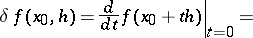(*)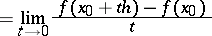on the assumption that it exists for all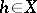. This is how the first variation was introduced by R. Gâteaux in 1913–1914. This definition for functionals of the classical calculus of variations was given by J.L. Lagrange (cf. Variation of a functional).

The expression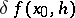need not necessarily be a linear functional in, but it is always a homogeneous function of the first degree in. The mapping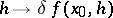is also known as the Gâteaux differential or weak differential. Beginning with the work of P. Lévy, it is usual to stipulate the linearity and continuity ofin: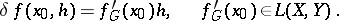where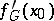is called the Gâteaux derivative. Second, etc., variations are defined similarly to (*). See also Variation; Second variation; Differentiation of a mapping.